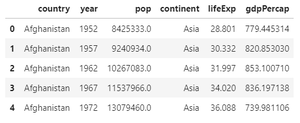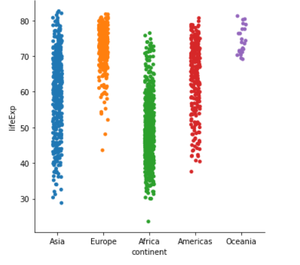Open In App
Related Articles
• Write an Interview Experience

# Python Seaborn – Strip plot illustration using Catplot

Seaborn is a data visualization library that is based on matplotlib. A high-level interface is provided by seaborn for drawing informative and attractive statistical graphics. Seaborn Catplot is a new addition to seaborn that makes plotting easier and involves categorical variables. It is used to show the relation between categorical variables like strip plot, box plot and so more with numerical variables.

Stripplot which is represented by strip is the default illustration in catplot().

Step-by-step Approach:

• Import required modules.

## Python3

 `# importing modules``import` `pandas as pnd``import` `matplotlib.pyplot as plt``import` `seaborn as sbn`

• Assign dataset and convert it into a data frame.

## Python3

 `# fetching data from the url``url_data``=``'http://bit.ly/2cLzoxH'` `# using pandas for reading the data file``# storing the data in input_data variable``input_data``=``pnd.read_csv(url_data)`

• Display data frame.

## Python3

 `# head() function helps to see the first n rows of data``# by default n is 5 in head function``input_data.head(``5``)`

Output:• Finally, depict the illustration.

## Python3

 `# using seaborn module to show the relation between``# categorical variables and numerical variables``sbn.catplot(x``=``'continent'``, y``=``'lifeExp'``, data``=``input_data)`

Output:Below is the complete program based on the above approach:

## Python3

 `# importing modules``import` `pandas as pnd``import` `matplotlib.pyplot as plt``import` `seaborn as sbn`   `# fetching data from the url``url_data``=``'http://bit.ly/2cLzoxH'` `# using pandas for reading the data file``# storing the data in input_data variable``input_data``=``pnd.read_csv(url_data)`   `# using seaborn module to show the relation between``# categorical variables and numerical variables``sbn.catplot(x``=``'continent'``, y``=``'lifeExp'``, data``=``input_data)`

Output: